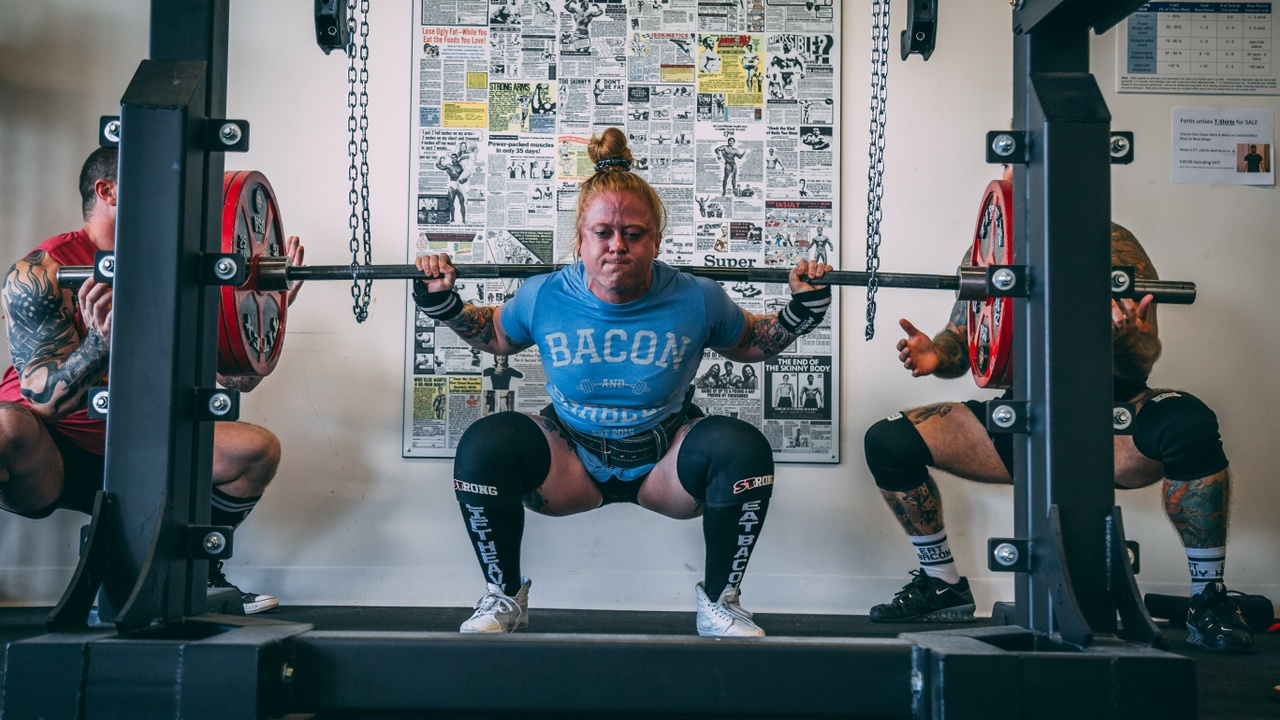# CSCS Calculations | How to Calculate Force, Work, and Power During a Barbell Squat

Aug 11, 2022Photo Credit: Alora Griffiths

The beauty of program design is that we can create a program to improve the stated or sport-specific needs of our athletes. The goal of our needs analysis is to understand where the opportunities lie so that we can customize and individualize our approach.

Following 1RM testing, you might be wondering how further to break down the specific components of these lifts to help you decide on training variables and program manipulation.

The three components of 1RM testing include force output, amount of work done, and amount of power.

#### Force = Mass x Acceleration

Force is calculated as mass times the acceleration of gravity with the barbell traveling at a constant velocity (to keep it simple). Keep in mind, the acceleration of gravy is 9.8m/s2

Let's say an athlete did a 100kg back squat x 9.8m/s2 = 980 N (Newtons)

Keep in mind that the acceleration isn't always constant, so we'll use 0.8m/s2 to demonstrate this.

100kg back squat x (9.8m/s2 + 0.8m/s2

100kg back squat x 10.6m/s2 = 1060 N (Newtons)

Keep in mind this downward force, so we have to apply the downward force, plus the upward force that would equalize it on the way up, plus the acceleration to speed the bar up faster (if needed) on the concentric portion of the back squat.

#### Work = Force x Displacement

Work is calculated as force times displacement.

= 980 N (the Force from above)

Let's assume the athlete is displacing the bar when doing the back squat by 1 meter.

980 N x 1 M = 980 Newton Meters or Jules

= 980 J

If you're wondering why we have to calculate work in order to calculate power it's because you can't directly calculate from force to power, so work serves as an intermediary to get to power calculations.

#### Power = Work/Time

Power is calculated as work divided by time.

= 980 J

• One athlete does a 2-second concentric portion of the barbell back squat (faster up)
• A second athlete does a 4-second grinding rep portion of the back squat (slower up)

Athlete 1:

• = 980 J/2 seconds = 490 Watts of Power

Athlete 2:

• 980J/4 seconds = 245 Watts

So pushing the bar up in 2 seconds compared to 4 seconds doubles the power output. So, there are a number of training variables that we can adjust in order to promote power adaptations. For example, giving our athletes a tempo back squat where they are increasing loads under a 2-second concentric portion of the lift should improve their ability to move more force faster to create more power output.

Once you know where the opportunities lie for force and power, you'll be able to prioritize your training program variables more easily.

#### Support & Courses Available

Ready for even more support? Our Program Design 101 Course teaches you exactly how to organize an annual training plan, how to optimize different training adaptations, and even provides sport-specific examples and even includes done for you programming templates by phase. Click the link here to check it out.